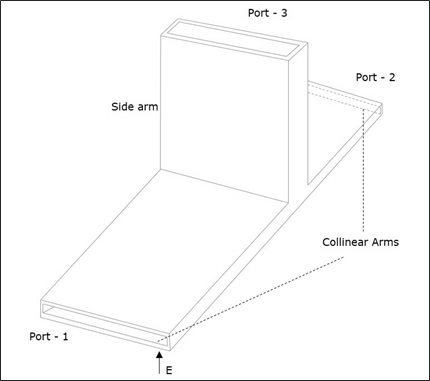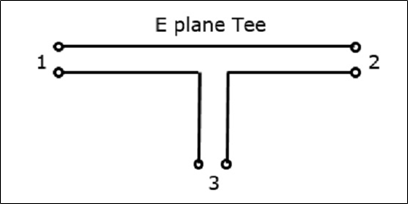# Microwave Engineering - E-Plane Tee

An E-Plane Tee junction is formed by attaching a simple waveguide to the broader dimension of a rectangular waveguide, which already has two ports. The arms of rectangular waveguides make two ports called collinear ports i.e., Port1 and Port2, while the new one, Port3 is called as Side arm or E-arm. T his E-plane Tee is also called as Series Tee.

As the axis of the side arm is parallel to the electric field, this junction is called E-Plane Tee junction. This is also called as Voltage or Series junction. The ports 1 and 2 are 180° out of phase with each other. The cross-sectional details of E-plane tee can be understood by the following figure.The following figure shows the connection made by the sidearm to the bi-directional waveguide to form the parallel port.## Properties of E-Plane Tee

The properties of E-Plane Tee can be defined by its $[S]_{3x3}$ matrix.

It is a 3×3 matrix as there are 3 possible inputs and 3 possible outputs.

$[S] = \begin{bmatrix} S_{11}& S_{12}& S_{13}\\ S_{21}& S_{22}& S_{23}\\ S_{31}& S_{32}& S_{33} \end{bmatrix}$ ........ Equation 1

Scattering coefficients $S_{13}$ and $S_{23}$ are out of phase by 180° with an input at port 3.

$S_{23} = -S_{13}$........ Equation 2

The port is perfectly matched to the junction.

$S_{33} = 0$........ Equation 3

From the symmetric property,

$S_{ij} = S_{ji}$

$S_{12} = S_{21} \: \: S_{23} = S_{32} \: \: S_{13} = S_{31}$........ Equation 4

Considering equations 3 & 4, the $[S]$ matrix can be written as,

$[S] = \begin{bmatrix} S_{11}& S_{12}& S_{13}\\ S_{12}& S_{22}& -S_{13}\\ S_{13}& -S_{13}& 0 \end{bmatrix}$........ Equation 5

We can say that we have four unknowns, considering the symmetry property.

From the Unitary property

$$[S][S]\ast = [I]$$

$$\begin{bmatrix} S_{11}& S_{12}& S_{13}\\ S_{12}& S_{22}& -S_{13}\\ S_{13}& -S_{13}& 0 \end{bmatrix} \: \begin{bmatrix} S_{11}^{*}& S_{12}^{*}& S_{13}^{*}\\ S_{12}^{*}& S_{22}^{*}& -S_{13}^{*}\\ S_{13}^{*}& -S_{13}^{*}& 0 \end{bmatrix} = \begin{bmatrix} 1& 0& 0\\ 0& 1& 0\\ 0& 0& 1 \end{bmatrix}$$

Multiplying we get,

(Noting R as row and C as column)

$R_1C_1 : S_{11}S_{11}^{*} + S_{12}S_{12}^{*} + S_{13}S_{13}^{*} = 1$

$\left | S_{11} \right |^2 + \left | S_{11} \right |^2 + \left | S_{11} \right |^2 = 1$ ........ Equation 6

$R_2C_2 : \left | S_{12} \right |^2 + \left | S_{22} \right |^2 + \left | S_{13} \right |^2 = 1$ ......... Equation 7

$R_3C_3 : \left | S_{13} \right |^2 + \left | S_{13} \right |^2 = 1$ ......... Equation 8

$R_3C_1 : S_{13}S_{11}^{*} - S_{13}S_{12}^{*} = 1$ ......... Equation 9

Equating the equations 6 & 7, we get

$S_{11} = S_{22}$ ......... Equation 10

From Equation 8,

$2\left | S_{13} \right |^2 \quad or \quad S_{13} = \frac{1}{\sqrt{2}}$ ......... Equation 11

From Equation 9,

$S_{13}\left ( S_{11}^{*} - S_{12}^{*} \right )$

Or $S_{11} = S_{12} = S_{22}$ ......... Equation 12

Using the equations 10, 11, and 12 in the equation 6,

we get,

$\left | S_{11} \right |^2 + \left | S_{11} \right |^2 + \frac{1}{2} = 1$

$2\left | S_{11} \right |^2 = \frac{1}{2}$

Or $S_{11} = \frac{1}{2}$ ......... Equation 13

Substituting the values from the above equations in $[S]$ matrix,

We get,

$$\left [ S \right ] = \begin{bmatrix} \frac{1}{2}& \frac{1}{2}& \frac{1}{\sqrt{2}}\\ \frac{1}{2}& \frac{1}{2}& -\frac{1}{\sqrt{2}}\\ \frac{1}{\sqrt{2}}& -\frac{1}{\sqrt{2}}& 0 \end{bmatrix}$$

We know that $[b]$ = $[S] [a]$

$$\begin{bmatrix}b_1 \\ b_2 \\ b_3 \end{bmatrix} = \begin{bmatrix} \frac{1}{2}& \frac{1}{2}& \frac{1}{\sqrt{2}}\\ \frac{1}{2}& \frac{1}{2}& -\frac{1}{\sqrt{2}}\\ \frac{1}{\sqrt{2}}& -\frac{1}{\sqrt{2}}& 0 \end{bmatrix} \begin{bmatrix} a_1\\ a_2\\ a_3 \end{bmatrix}$$

This is the scattering matrix for E-Plane Tee, which explains its scattering properties.